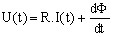# Solid conductor component

## Introduction

From the physical point of view, a solid conductor is a thick conductor, where the effect of the eddy currents must be considered. The skin depth has a value comparable to or smaller than the conductor cross-section, and the current density is non-uniform on the conductor cross-section.

## Solid conductor: representation

A solid conductor is described:

• in the finite elements domain by a region of solid conductor type
• in the electrical circuit by a component of solid conductor type

## Solid conductor: relation U, I, Φ

The basic relation linking the current I, the voltage U and the flux Φ is given below.

The current flowing through a solid conductor component is the total current I flowing through the correspondent solid conductor region of the finite element domain.

The voltage at the terminals of a solid conductor component is the voltage U between the front and the back faces of the modeled solid conductor region.where:

• R is the resistance of the conductor
• Φ is the flux embraced by the conductor